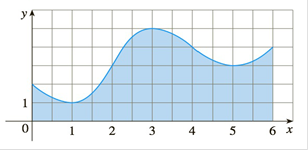Chapter 7.7, Problem 29E

Chapter
Section
Textbook Problem

# Estimate the area under the graph in the figure by using(a) the Trapezoidal Rule, (b) the Midpoint Rule, and(c) Simpson’s Rule, each with n = 6 .To determine

To evaluate: The area under the given graph by using.

(a) Trapezoidal rule.

(b) Midpoint rule.

(c) Simpson rule with n=6

Explanation

Formula used:

TA=i=0n1Δ2[f(a+iΔ)+f(a+(i+1)Δ)]

MA=i=0n1Δ[f(a+(i+12)Δ)]

SA=23MA+13TA

Kindly note that the Simpson’s formula given in the notebook can be simplified to SA=23MA+13TA

Calculation: The exact value of the function f(x) is

f(x)=06f(x)dx

b=6, a=0, n=6

Δ=1

By trapezoidal rule.

TA=12[f(x0)+2f(x1)+2f(x2)+2f(x3)+2f(x4)+2f(x5)+x6]=12[f(0)+2f(1)+2f(2)+2f(3)+2f(4)+2f(5)+f6]=12[2+2+6+10+8+8+6+4]=19

By Midpoint rule

### Still sussing out bartleby?

Check out a sample textbook solution.

See a sample solution

#### The Solution to Your Study Problems

Bartleby provides explanations to thousands of textbook problems written by our experts, many with advanced degrees!

Get Started

#### Expand each expression in Exercises 122. (y3+2y2+y)(y2+2y1)

Finite Mathematics and Applied Calculus (MindTap Course List)

#### A sample of n = 9 scores has X = 108. What is the sample mean?

Essentials of Statistics for The Behavioral Sciences (MindTap Course List)

#### In Exercises 4144, determine whether the statement is true or false. 42. 5 5

Applied Calculus for the Managerial, Life, and Social Sciences: A Brief Approach

#### True or False: If f′(x) = g′(x), then f(x) = g(x).

Study Guide for Stewart's Single Variable Calculus: Early Transcendentals, 8th

#### The equation of the tangent plane to for is:

Study Guide for Stewart's Multivariable Calculus, 8th